Block Ciphers (part 1)

# Modern Private Key Ciphers (part 1)

• now want to concentrate on modern encryption systems
• these usually consider the message as a sequence of bits
• (eg as a series of ASCII characters concatenated)
• have two broad families of methods
• stream ciphers and block ciphers

### Stream Ciphers and the Vernam cipher

• process the message bit by bit (as a stream)
• the most famous of these is the Vernam cipher
• (also known as the one-time pad)
• invented by Vernam, working for AT&T, in 1917
• simply add bits of message to random key bits
• need as many key bits as message, difficult in practise
• (ie distribute on a mag-tape or CDROM)
• is unconditionally secure provided key is truly random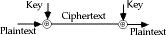• since difficult to distribute so much key, why not generate keystream from a smaller (base) key
• use some pseudo-random function to do this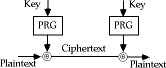• although this looks very attractive, it proves to be very very difficult in practise to find a good pseudo-random function that is cryptographically strong
• this is still an area of much research

### Block Ciphers

• in a block cipher the message is broken into blocks, each of which is then encrypted (ie like a substitution on very big characters - 64-bits or more)
• most modern ciphers we will study are of this form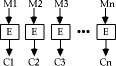### Shannons Theory of Secrecy Systems

• Claude Shannon wrote some of the pivotal papers on modern cryptology theory in 1949:
• C E Shannon, "Communication Theory of Secrecy Systems", Bell System Technical Journal, Vol 28, Oct 1949, pp 656-715
• C E Shannon, "Prediction and Entropy of printed English", Bell System Technical Journal, Vol 30, Jan 1951, pp 50-64
• in these he developed the concepts of:
• entropy of a message,
• redundancy in a language,
• theories about how much information is needed to break a cipher
• defined the concepts of computationally secure vs unconditionally secure ciphers
• he showed that the Vernam cipher is the only currently known unconditionally secure cipher, provided the key is truly random
• also showed that if try to encrypt English text by adding to other English text (ie a Bookcipher), this is not secure since English is 80% redundant, giving ciphertext with 60% redundancy, enough to break
• a similar technique can also be used if the same random key stream is used twice on different messages, the redundancy in the messages is sufficient to break this
• as discussed earlier, exhaustive key search is the most fundamental attack, and is directly proportional to the size of the key
• can tabulate these for reasonable assumptions about the number of operations possible (& parallel tests):
```Key Size (bits)         Time (1us/test)              Time (1us/106test)
24                      8.4 sec                      8.4 usec
32                      35.8 mins                    2.15 msec
40                      6.4 days                     550 msec
48                      4.46 yrs                     2.35 mins
56                      ~2000 yrs                    10.0 hrs
64                      ~500000 yrs                  107 days

```
• as the ultimate limit, it can be shown from energy consumption considerations that the maximum number of possible elementary operations in 1000 years is about: 3 x 10 ^(48)
• similarly can show that if need say 10 atoms to store a bit of information, then the greatest possible number of bits storable in a volume of say the moon is: 10 ^(45)
• if a cipher requires more operations, or needs more storage than this, it is pretty reasonable to say it is computationally secure
• eg to test all possible 128-bit keys in Lucifer takes about 3 x 10 ^(48) encryptions, needing 10 ^(19) years

### Substitution-Permutation Ciphers

• in his 1949 paper Shannon also introduced the idea of substitution-permutation (S-P) networks, which now form the basis of modern block ciphers
• an S-P network is the modern form of a substitution-transposition product cipher
• S-P networks are based on the two primitive cryptographic operations we have seen before
Substitution Operation
• a binary word is replaced by some other binary word
• the whole substitution function forms the key
• if use n bit words, the key is 2^(n)!bits, grows rapidly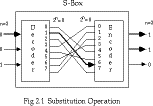• can also think of this as a large lookup table, with n address lines (hence 2^(n) addresses), each n bits wide being the output value
• will call them S-boxes
Permutation Operation
• a binary word has its bits reordered (permuted)
• the re-ordering forms the key
• if use n bit words, the key is n!bits, which grows more slowly, and hence is less secure than substitution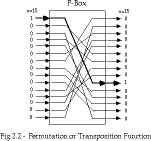• this is equivalent to a wire-crossing in practise (though is much harder to do in software)
• will call these P-boxes
Substitution-Permutation Network
• Shannon combined these two primitives
• he called these mixing transformations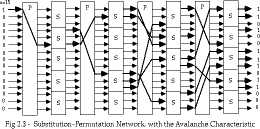• Shannons mixing transformations are a special form of product ciphers where
S-Boxes
provide confusion of input bits
P-Boxes
provide diffusion across S-box inputs
• in general these provide the following results, as described in:
A F Webster & S E Tavares "On the Design of S-boxes", in Advances in Cryptology - Crypto 85, Lecture Notes in Computer Science, No 218, Springer-Verlag, 1985, pp 523-534

Avalanche effect

• where changing one input bit results in changes of approx half the output bits

More formally, a function f has a good avalanche effect if for each bit i,0<=i<m, if the 2^(m) plaintext vectors are divided into 2^(m-1) pairs X and X_(i) with each pair differing only in bit i; and if the 2^(m-1) exclusive-or sums, termed avalanche vectors

V_(i) = f(X) (+) f(X_(i))

are compared, then about half of these sums should be found to be 1.

Completeness effect

• where each output bit is a complex function of all the input bits
More formally, a function f has a good completeness effect if for each bit j,0<=j<m, in the ciphertext output vector, there is at least one pair of plaintext vectors X and X_(i) which differ only in bit i, and for which f(X) and f(X_(i)) differ in bit j

Practical Substitution-Permutation Networks

• in practise we need to be able to decrypt messages, as well as to encrypt them, hence either:
• have to define inverses for each of our S & P-boxes, but this doubles the code/hardware needed, or
• define a structure that is easy to reverse, so can use basically the same code or hardware for both encryption and decryption
• Horst Feistel, working at IBM Thomas J Watson Research Labs devised just such a structure in early 70's, which we now call a feistel cipher
• the idea is to partition the input block into two halves, L(i-1)and R(i-1), and use only R(i-1)in each round i (part) of the cipher
• the function g incorporates one stage of the S-P network, controlled by part of the key K(i)known as the ith subkey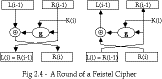• this can be described functionally as:
L(i) = R(i-1)

R(i) = L(i-1) (+) g(K(i), R(i-1))

• this can easily be reversed as seen in the above diagram, working backwards through the rounds
• in practise link a number of these stages together (typically 16 rounds) to form the full cipher

### Lucifer

• Lucifer is the first publically known example of a practical substitution-permutation cipher
• it was developed by Horst Feistel at IBM labs
• the best known public reference is his paper:
• Horst Feistel, "Cryptography and Computer Privacy", Scientific American, Vol 228(5), May 1973, pp 15-23.
• it provides an overview of his work, but actual details of Lucifer are very sketchy, the best detailed reference:
• Arthur Sorkin, "Lucifer, A Cryptographic Algorithm", Cryptologia, Vol 8(1), Jan 1984, pp 22-41, with addenda in Vol 8(3) pp260-261
• this contains a detailed description of the algorithm, plus a Fortran implementation
• the original descriptions are not the clearest

Sorkin Fig 1 p24

• redrawn in modern form, Lucifer has the following form: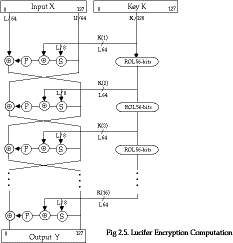• Lucifer uses 128-bit data blocks and 128-bit keys, and uses a Feistel structure
• the subkeys used in each round are taken from the left part of the key, which is then rotated left 56-bits, so all key bits are used
• the S-P function for Lucifer has the following structure:
• the substitution consists of 8 identical pairs of 4-bit S-boxes (S0 & S1) used in alternate order (S0|S1) or (S1|S0) depending on the key
• then the subkey is added modulo 2
• and the result is permuted through a combination of small 8-bit permutations and a large 128-bit simple permutation (convolution)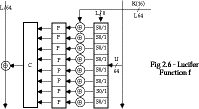• for details see Sorkin
• from the little known discussion, Lucifer appears to be a fairly well constructed cipher, although recently it has been theorectically broken by differential cryptanalysis
• its main claim is that it is the predecessor the DES, developed by IBM in the mid 70's and submitted to the NBS (& NSA) to become the standard encryption algorithm for commercial use at the time

### DES

• in May 1973, and again in Aug 1974 the NBS (now NIST) called for possible encryption algorithms for use in unclassified government applications
• response was mostly disappointing, however IBM submitted their Lucifer design
• following a period of redesign and comment it became the Data Encryption Standard (DES)
• it was adopted as a (US) federal standard in Nov 76, published by NBS as a hardware only scheme in Jan 77 and by ANSI for both hardware and software standards in ANSI X3.92-1981 (also X3.106-1983 modes of use)
• subsequently it has been widely adopted and is now published in many standards around the world
• cf Australian Standard AS2805.5-1985
• one of the largest users of the DES is the banking industry, particularly with EFT, and EFTPOS
• it is for this use that the DES has primarily been standardised, with ANSI having twice reconfirmed its recommended use for 5 year periods - a further extension is not expected however
• although the standard is public, the design criteria used are classified and have yet to be released
• there has been considerable controversy over the design, particularly in the choice of a 56-bit key
• W Diffie, M Hellman "Exhaustive Cryptanalysis of the NBS Data Encryption Standard" IEEE Computer 10(6), June 1977, pp74-84
• M Hellman "DES will be totally insecure within ten years" IEEE Spectrum 16(7), Jul 1979, pp 31-41
• recent analysis has shown despite this that the choice was appropriate, and that DES is well designed
• rapid advances in computing speed though have rendered the 56 bit key susceptible to exhaustive key search, as predicted by Diffie & Hellman
• the DES has also been theorectically broken using a method called Differential Cryptanalysis, however in practise this is unlikely to be a problem (yet)

#### Overview of the DES Encryption Algorithm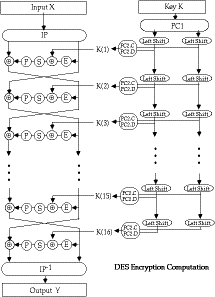• the basic process in enciphering a 64-bit data block using the DES consists of:
• an initial permutation (IP)
• 16 rounds of a complex key dependent calculation f
• a final permutation, being the inverse of IP
• in more detail the 16 rounds of f consist of: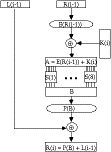• this can be described functionally as
L(i) = R(i-1)

R(i) = L(i-1) (+) P(S( E(R(i-1))(+) K(i) ))

and forms one round in an S-P network

• the subkeys used by the 16 rounds are formed by the key schedule which consists of:
• an initial permutation of the key (PC1) which selects 56-bits in two 28-bit halves
• 16 stages consisting of
• selecting 24-bits from each half and permuting them by PC2 for use in function f,
• rotating each half either 1 or 2 places depending on the key rotation schedule KS
• this can be described functionally as:
K(i) = PC2(KS(PC1(K),i))
• the key rotation schedule KS is specified as:
```   Round       1    2   3    4    5    6    7    8    9    10   11   12   13   14   15   16
KS        1    1   2    2    2    2    2    2    1    2     2    2   2    2    2    1
Total Rot     1    2   4    6    8    10   12   14   15   17   19   21   23   25   27   28

```
• more details on the various DES functions can be found in your textbooks
• following is a walk-through of a DES encryption calculation taken from:
H Katzan, "The Standard Data Encryption Algorithm", Petrocelli Books, New York, 1977

### DES Modes of Use

• DES encrypts 64-bit blocks of data, using a 56-bit key
• we need some way of specifying how to use it in practise, given that we usually have an arbitrary amount of information to encrypt
• the way we use a block cipher is called its Mode of Use and four have been defined for the DES by ANSI in the standard: ANSI X3.106-1983 Modes of Use)
• modes are either:
Block Modes
splits messages in blocks (ECB, CBC)
Stream Modes
on bit stream messages (CFB, OFB)

#### Block Modes

Electronic Codebook Book (ECB)
- where the message is broken into independent 64-bit blocks which are encrypted
```			C_(i) = DES_(K1) (P_(i))
```
Cipher Block Chaining (CBC)
- again the message is broken into 64-bit blocks, but they are linked together in the encryption operation with an IV
C_(i) = DES_(K1) (P_(i)(+)C_(i-1)) C_(-1)=IV

#### Stream Modes

Cipher FeedBack (CFB)
- where the message is treated as a stream of bits, added to the output of the DES, with the result being feed back for the next stage
C_(i) = P_(i)(+) DES_(K1) (C_(i-1)) C_(-1)=IV
Output FeedBack (OFB)
- where the message is treated as a stream of bits, added to the message, but with the feedback being independent of the message
C_(i) = P_(i)(+) O_(i) O_(i) = DES_(K1)(O_(i-1)) O_(-1)=IV
• []

### Limitiations of Various Modes

#### ECB

• repetitions in message can be reflected in ciphertext
• if aligned with message block
• particularly with data such graphics
• or with messages that change very little, which become a code-book analysis problem
• weakness is because enciphered message blocks are independent of each other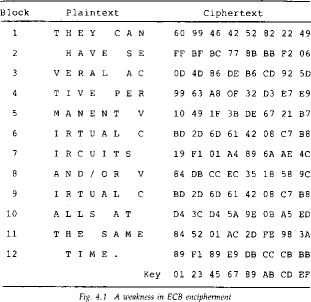Davies Fig 4.1 p81

#### CBC

• use result of one encryption to modify input of next
• hence each ciphertext block is dependent on all message blocks before it
• thus a change in the message affects the ciphertext block after the change as well as the original block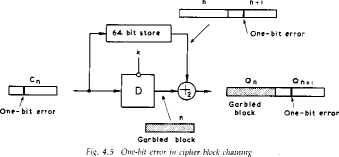Davies Fig 4.5 p85

• to start need an Initial Value (IV) which must be known by both sender and receiver
• however if IV is sent in the clear, an attacker can change bits of the first block, and change IV to compensate
• hence either IV must be a fixed value (as in EFTPOS) or it must be sent encrypted in ECB mode before rest of message
• also at the end of the message, have to handle a possible last short block
• either pad last block (possible with count of pad size), or use some fiddling to double up last two blocks
• see Davies for examples

#### CFB

• when data is bit or byte oriented, want to operate on it at that level, so use a stream mode
• the block cipher is use in encryption mode at both ends, with input being a feed-back copy of the ciphertext
• can vary the number of bits feed back, trading off efficiency for ease of use
• again errors propogate for several blocks after the error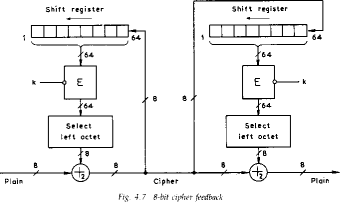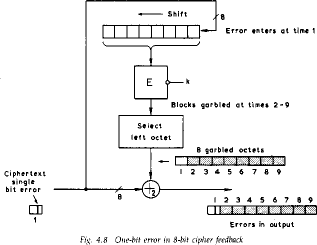Davies Fig 4.7 p88 & Fig 4.8 p89

#### OFB

• also a stream mode, but intended for use where the error feedback is a problem, or where the encryptions want to be done before the message is available
• is superficially similar to CFB, but the feedback is from the output of the block cipher and is independent of the message, a variation of a Vernam cipher
• again an IV is needed
• sender and receiver must remain in sync, and some recovery method is needed to ensure this occurs
• although originally specified with varying m-bit feedback in the standards, subsequent research has shown that only 64-bit OFB should ever be used (and this is the most efficient use anyway), see
D Davies, G Parkin, "The Average Cycle Size of the Key Stream in Output Feedback Encipherment" in Advances in Cryptology - Crypto 82, Plenum Press, 1982, pp97-98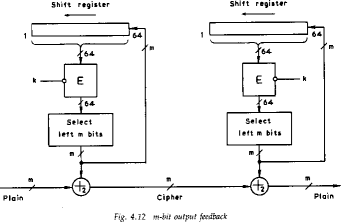Davies Fig 4.12 p94

### DES Weak Keys

• with many block ciphers there are some keys that should be avoided, because of reduced cipher complexity
• these keys are such that the same sub-key is generated in more than one round, and they include:

#### Weak Keys

• he same sub-key is generated for every round
• DES has 4 weak keys

#### Semi-Weak Keys

• only two sub-keys are generated on alternate rounds
• DES has 12 of these (in 6 pairs)

#### Demi-Semi Weak Keys

• have four sub-keys generated
• none of these cause a problem since they are a tiny fraction of all available keys
• however they MUST be avoided by any key generation program 

### DES Design Principles

• although the standard for DES is public, the design criteria used are classified and have yet to be released
• some information is known, and more has been deduced
L P Brown, "A Proposed Design for an Extended DES", in Computer Security in the Age of Information, W. J. Caelli (ed), North-Holland, pp 9-22, 1989

L P Brown, J R Seberry, "On the Design of Permutation Boxes in DES Type Cryptosystems", in Advances in Cryptology - Eurocrypt '89, Lecture Notes in Computer Science, vol 434, pp 696-705, J.J. Quisquater, J. Vanderwalle (eds), Springer-Verlag, Berlin, 1990.

L P Brown and J R Seberry, "Key Scheduling in DES Type Cryptosystems," in Advances in Cryptology - Auscrypt '90, Lecture Notes in Computer Science, vol 453, pp 221-228, J. Seberry, J. Pieprzyk (eds), Springer-Verlag, Berlin, 1990.

• will briefly overview the basic results, for more detailed analyses see the above papers

#### DES S-Box Design Criteria

• each S-box may be considered as four substitution functions
• these 1-1 functions map inputs 2,3,4,5 onto output bits
• a particular function is selected by bits 1,6
• this provides an autoclave feature

#### DES Design Criteria

• there were 12 criterion used, resulting in about 1000
• possible S-Boxes, of which the implementers chose 8
• these criteria are CLASSIFIED SECRET
• however, some of them have become known
• The following are design criterion:
R1: Each row of an S-box is a permutation of 0 to 15

R2: No S-Box is a linear of affine function of the input

R3: Changing one input bit to an S-box results in changing at least two output bits

R4: S(x) and S(x+001100) must differ in at least 2 bits

• The following are said to be caused by design criteria
R5: S(x) [[pi]] S(x+11ef 00) for any choice of e and f

R6: The S-boxes were chosen to minimize the difference between the number of 1's and 0's in any S-box output when any single input is held constant

R7: The S-boxes chosen require significantly more minterms than a random choice would require

Meyer Tables 3-17, 3-18

#### DES Permutation Tables

• there are 5 Permutations used in DES:
• IP and IP^(-1) , P, E, PC1, PC2
• their design criteria are CLASSIFIED SECRET
• it has been noted that IP and IP^(-1) and PC1 serve no cryptological function when DES is used in ECB or CBC modes, since searches may be done in the space generated after they have been applied
• E, P, and PC2 combined with the S-Boxes must supply the required dependence of the output bits on the input bits and key bits (avalanche and completeness effects)

#### Ciphertext Dependence on Input and Key

• the role of P, E, and PC2 is distribute the outputs of the S-boxes so that each output bit becomes a function of all the input bits in as few rounds as possible
• Carl Meyer (in Meyer 1978, or Meyer & Matyas 1982) performed this analysis on the current DES design
##### Ciphertext dependence on Plaintext
• define G_(i,j) a 64*64 array which shows the dependence of output bits X(j) on input bits X(i)
• examine G_(0,j) to determine how fast complete dependence is achieved
• to build G_(0,1) use the following
L(i) = R(i-1)

R(i) = L(i-1) (+) f( K(i), R(i-1))

• DES P reaches complete dependence after 5 rounds
• []
##### Ciphertext dependence on Key
• Carl Meyer also performed this analysis
• define F_(i,j) a 64*56 array which shows the dependence of output bits X(j) on key bits U(i) (after PC1 is used)
• examine F_(0,j) to determine how fast complete dependence is achieved
• DES PC2 reaches complete dependence after 5 rounds

#### Key Scheduling and PC2

• Key Schedule
• is a critical component in the design
• must provide different keys for each round otherwise security may be compromized (see Grossman & Tuckerman 1978)
• current scheme can result in weak keys which give the same, 2 or 4 keys over the 16 rounds
• Key Schedule and PC-2 Design
• is performed in two 28-bit independent halves
• C-side provides keys to S-boxes 1 to 4
• D-side provides keys to S-boxes 5 to 8
• the rotations are used to present different bits of the key for selection on successive rounds
• PC-2 selects key-bits and distributes them over the S-box inputs

### Possible Techniques for Improving DES

• multiple enciphering with DES
• extending DES to 128-bit data paths and 112-bit keys
• extending the Key Expansion calculation

### Triple DES

• DES variant
• standardised in ANSI X9.17 & ISO 8732 and in PEM for key management
• proposed for general EFT standard by ANSI X9
• backwards compatible with many DES schemes
• uses 2 or 3 keys
```			C = DES_(K1) Bbc{(DES^(-1)_(K2)Bbc{(DES_(K1)(P)))
```
• no known practical attacks
• brute force search impossible
• meet-in-the-middle attacks need 2^(56) PC pairs per key
• popular current alternative

 follow with Modes of Use illustrations
 follow with Appendix A from AS2805.5 (also found in Seberry Appendix B2)
 follow with Meyer - Fig 3-17; Fig 3-15, 3-16, 3-19; Fig 3-18, 3-21.
[CSC Info]